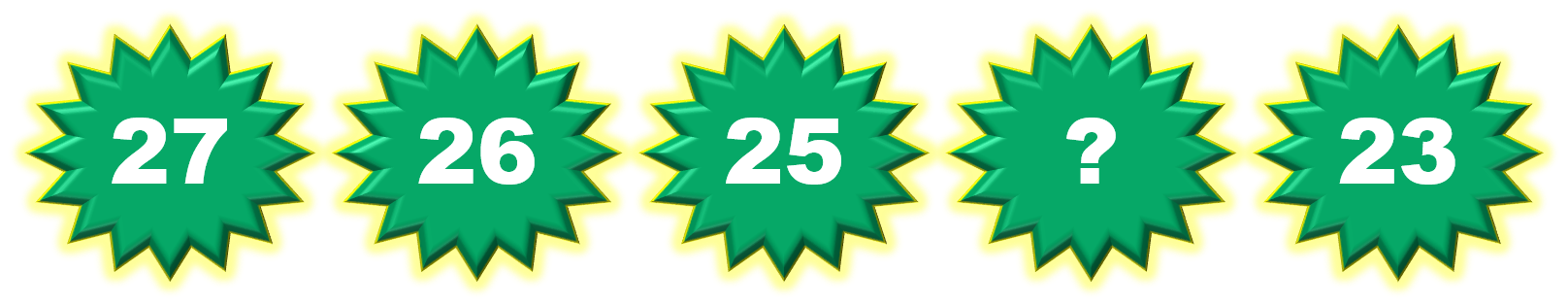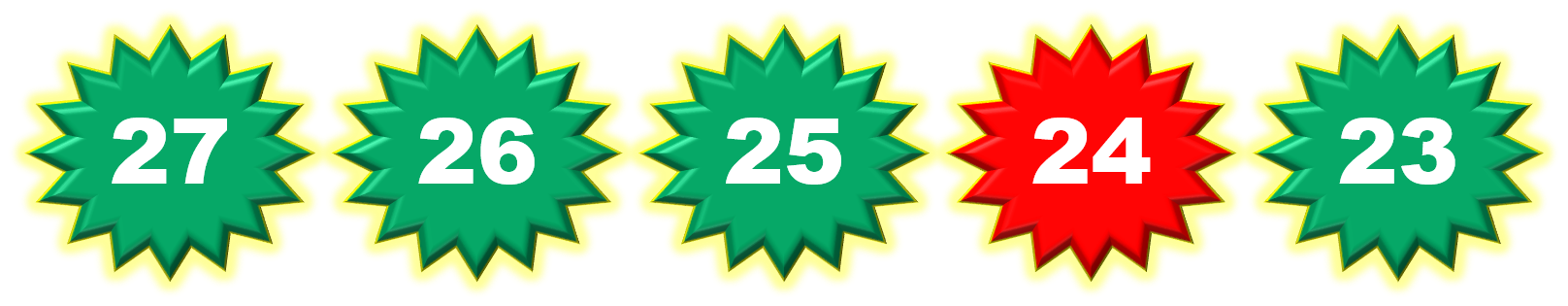1
visibility

Consider the given number pattern and identify the missing number.• A

24

• B

42

• C

32

• D

21

The correct answer is 24.

Solution:

Considering the given number pattern.Here, the numbers are decreasing.
So, to find the missing number, we first find the rule.

Note that:
27 - 1 = 26
26 - 1 = 25
25 - 1 = 24
24 - 1 = 23

So, the rule is "subtract 1".Then, the missing number is 24.